Next: Worked example 12.3: Circular Up: Orbital motion Previous: Worked example 12.1: Gravity

## Worked example 12.2: Acceleration of a rocket

Question: A rocket is located a distance 3.5 times the radius of the Earth above the Earth's surface. What is the rocket's free-fall acceleration?

Answer: Let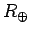be the Earth's radius. The distance of the rocket from the centre of the Earth is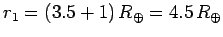. We know that the free-fall acceleration of the rocket when its distance from the Earth's centre is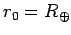(i.e., when it is at the Earth's surface) is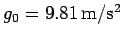. Moreover, we know that gravity is an inverse-square law (i.e.,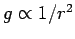). Hence, the rocket's acceleration is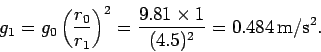Richard Fitzpatrick 2006-02-02